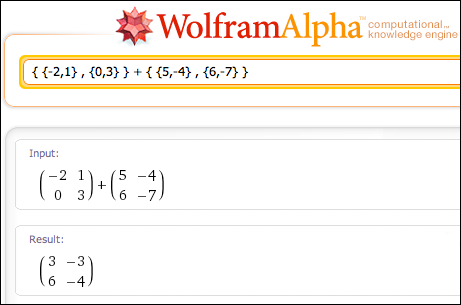﻿ Basic Arithmetic With Matrices
BASIC ARITHMETIC WITH MATRICES

Whenever you get a new mathematical object (like matrices),
it's important to develop tools to work with the new object.

In this exercise, you'll learn how to do basic arithmetic operations with matrices:
adding, subtracting, and multiplying by a constant.

Matrices can only be added or subtracted when they have the same size.
In this situation, you just add/subtract the corresponding entries.

For example, $$\begin{bmatrix} -2 & 1\cr 0 & 3 \end{bmatrix} + \begin{bmatrix} 5 & -4\cr 6 & -7 \end{bmatrix} = \begin{bmatrix} -2+5 & 1+(-4)\cr 0+6 & 3+(-7) \end{bmatrix} = \begin{bmatrix} 3 & -3\cr 6 & -4 \end{bmatrix}$$ $$\begin{bmatrix} -2 & 1\cr 0 & 3 \end{bmatrix} - \begin{bmatrix} 5 & -4\cr 6 & -7 \end{bmatrix} = \begin{bmatrix} -2-5 & 1-(-4)\cr 0-6 & 3-(-7) \end{bmatrix} = \begin{bmatrix} -7 & 5\cr -6 & 10 \end{bmatrix}$$

Precisely, we have:

Suppose matrices $\,A\,$ and $\,B\,$ have the same size.

Then, the sum $\,S=A+B\,$ is defined by: $$s_{ij} = a_{ij} + b_{ij}$$ The difference $\,D=A-B\,$ is defined by: $$d_{ij} = a_{ij} - b_{ij}$$ In particular, matrices with different sizes cannot be added or subtracted.

WolframAlpha uses braces $\,\{\ \}\,$ to input matrices, not brackets $\,[\ ]\,$.
Then, it displays the matrices (so you can verify your input) using parentheses $\,(\ )\,$.
Get used to this—different academic disciplines and different environments often use different notation.

The screenshot below shows how to input matrices at wolframalpha.com.
Each row also goes inside a pair of braces $\,\{\ \}\,$, with elements separated by commas, like this:   $\,\{-2,1\}\,$
The rows themselves are also separated by commas, like this:   $\,\{-2,1\}\ ,\ \{0,3\}\,$
The entire matrix is enclosed within a pair of braces, like this:   $\,\ \{\ \{-2,1\}\ ,\ \{0,3\}\ \}\,$
If you want, cut-and-paste the following input to WolframAlpha to duplicate what you see in the image below:
{ {-2,1} , {0,3} } + { {5,-4} , {6,-7} }Multiplying a Matrix by a Constant

It is equally easy to multiply a matrix by a constant; each entry gets multiplied by the constant.

For example, $$7 \begin{bmatrix} -2 & 1\cr 0 & 3 \end{bmatrix} = \begin{bmatrix} 7(-2) & 7(1)\cr 7(0) & 7(3) \end{bmatrix} = \begin{bmatrix} -14 & 7\cr 0 & 21 \end{bmatrix}$$

Precisely, we have:

DEFINITION multiplying a matrix by a constant
Let $\,M\,$ be a matrix, with members $\,m_{ij}\,$.
Let $\,k\,$ be a real number.

Then, the new matrix $\,kM\,$ has the same size as $\,M\,$;
the member in row $\,i\,$ and column $\,j\,$ of $\ kM\$ is $\ k\,m_{ij}\$.

Note: The real number multiplier is often called a constant or a scalar.

When working with matrices, it's important to distinguish between the real number $\,0\,$ and a zero matrix.
To help with this distinction, we define $\,0_{m\times n}\,$ (zero, with a subscript of $\,m\times n\,$)
to mean the zero matrix of size $\,m\times n\,$.

You can read $\,0_{m\times n}\,$ aloud as ‘the $\,m\,$ by $\,n\,$ zero matrix’.

Thus, if $\,A\,$ is a $\,2\times 3\,$ matrix, then: $$A-A= 0_{2\times 3}$$ Or, if $\,A\,$ is a $\,p\times q\,$ matrix, then: $$0A= 0_{p\times q}$$

Be aware that many advanced textbooks write simple things like $\,A-A=0,\,$
knowing that the audience has enough mathematical maturity to realize that the zero is really the zero matrix with the same size as $\,A\,$.
However, in this exercise, we will be careful to distinguish between the real number zero, and a zero matrix.

EXAMPLES:
Let   $A = \left[ \begin{smallmatrix} 2 & -1\cr 0 & 3\end{smallmatrix} \right] \,$,   $B = \left[ \begin{smallmatrix} -3 & -5\cr 1 & 0\end{smallmatrix} \right] \,$,   and   $C = \left[ \begin{smallmatrix} 0 & 1 & 1\cr 2 & -1 & 0\end{smallmatrix} \right] \,$.

Then,

\begin{align} A+2B &= \begin{bmatrix} 2 & -1\cr 0 & 3 \end{bmatrix} + 2 \begin{bmatrix} -3 & -5\cr 1 & 0 \end{bmatrix} \cr\cr &= \begin{bmatrix} 2 & -1\cr 0 & 3 \end{bmatrix} + \begin{bmatrix} -6 & -10\cr 2 & 0 \end{bmatrix} \cr\cr &= \begin{bmatrix} -4 & -11\cr 2 & 3 \end{bmatrix} \end{align}

As a second example, $\,A+C\,$ is not defined, since $\,A\,$ and $\,C\,$ have different sizes.
Master the ideas from this section